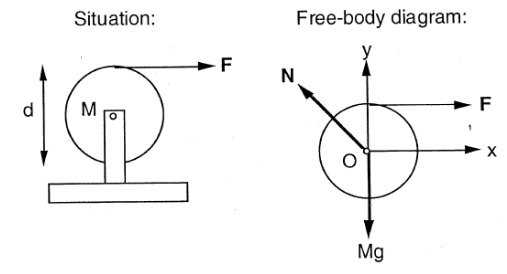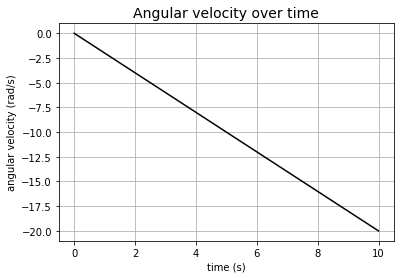# Rotational Motion#

Mechanics

## Angular Momentum Theorem#

From Newton’s second law:

$\vec{F}=ma=m\frac{d\vec{v}}{dt}=\frac{d(m\vec{v})}{dt}$

The product $$m\vec{v}$$ is called linear momentum, written as $$\vec{p}$$.

Taking the cross product of both sides with the position vector $$\vec{r}$$:

$\vec{r}\times\vec{F} = \vec{r}\times\left(\frac{d\vec{p}}{dt}\right)$

The product $$\vec{r}\times\vec{F}$$ is called the torque, denoted by $$\vec{\tau}$$. From the rules of cross product, the magnitude of $$\vec{\tau}$$ is given by:

$|\tau|=rF_\theta$

where $$F_\theta$$ is the tangential component of $$\vec{F}$$.

The direction of $$\vec{\tau}$$ is perpendicular to the plane formed by $$r$$ and $$F$$.

Therefore:

$\vec{\tau}=\vec{r}\times\frac{d\vec{p}}{dt}$

From the product rule of differentiation:

$\frac{d(\vec{r}\times\vec{p})}{dt}=\vec{r}\times\frac{d\vec{p}}{dt}+\frac{d\vec{r}}{dt}\times\vec{p}$

Since $$\frac{d\vec{r}}{dt}=\vec{v}$$ and $$m\vec{v}=\vec{p}$$, RHS in the equation above becomes:

$\vec{v}\times(m\vec{v})=m(\vec{v}\times\vec{v})=0$

Thus:

$\vec{\tau}=\frac{d(\vec{r}\times\vec{p})}{dt}$

The product $$\vec{r}\times\vec{p}$$ is defined as the angular momentum $$\vec{L}$$, and this is the angular momentum theorem:

$\vec{\tau}=\frac{d\vec{L}}{dt}$

## Moment of inertia#

The moment of inertia of a body $$I$$ composed of $$N$$ discrete sub-bodies is defined as:

$I=\sum_{i=1}^{N}m_ir_i^2$

Where $$m_i$$ is the mass of each sub-bodies, and $$r_i$$ is the distance of those sub-bodies from the axis of rotation.

With this definition, the angular momentum equation reduces to:

$\vec{\tau}=I\frac{d^2\theta}{dt^2}$

where $$\frac{d^2\theta}{dt^2}$$ represents angular acceleration.

For a continuous body, its moment of inertia $$I$$ is defined as:

$I=\int r^2dm=\int\rho(r)r^2dV$

## Tutorial Problem 4.5#

A cable is wrapped several times around a uniform, solid circular cylinder that can rotate about its axis. The cylinder has radius $$R$$, and mass $$m$$. The cable is pulled with a force of magnitude $$F$$. Assuming that the cable unwinds without stretching or slipping, what will be the angular acceleration of the cylinder?

Ignore the weight of the cable.import numpy as np
%matplotlib inline
import matplotlib.pyplot as plt
from matplotlib import animation, rc
from IPython.display import HTML
from sympy import Symbol, integrate

F = Symbol('F') # force
M = Symbol('M') # mass of cylinder
R = Symbol('R') # distance from the centre of the cylinder to where the force is applied
t = Symbol('t') # time

# x component of N = -F
# y component of N = Mg
# only F contributes to net torque
# sub into angular momentum equation
# for cylinder, I=MR**2/2

angular_acceleration = -(2*F)/(M*R)

angular_velocity = integrate(angular_acceleration, t)

angle = integrate(angular_velocity, t)

print("angular acceleration = ", angular_acceleration)

print("angular velocity = ", angular_velocity)

print("angle = ", angle)

angular acceleration =  -2*F/(M*R)
angular velocity =  -2*F*t/(M*R)
angle =  -F*t**2/(M*R)

# substitute arbitrary numbers
F = 10 # N
M = 10 # kg
R = 1 # m

t = np.linspace(0, 10, 500) # create list of time from 0 to 10 seconds

a_a = -(2*F)/(M*R) # angular acceleration

a_v = -2 * F * t / (M * R) # angular velocity

a = -(F * t**2) / (M * R) # angle

print("Angular acceleration = %.2f rad/s2" % (a_a))

X = R * np.cos(a) # x coordinate

Y = R * np.sin(a) # y coordinate

length = R * (-a / (2 * np.pi)) # length of string

l = np.zeros((len(t), len(t))) # store data in matrix to make animation
for i in range(len(t)):
for j in range(i+1):
l[i][j] = length[j]

# plot angular velocity over time

fig = plt.figure(figsize=(6,4))
plt.plot(t, a_v, 'k')
plt.xlabel('time (s)')
plt.title('Angular velocity over time', fontsize=14)
plt.grid(True)
plt.show()

Angular acceleration = -2.00 rad/s2nframes = len(t)

# Plot background axes
fig, ax = plt.subplots(figsize=(10,2))

# plot lines
line1, = ax.plot([], [], 'ro', lw=2)
line2, = ax.plot([], [], 'k', lw=0.5)
line3, = ax.plot([], [], 'k', lw=2)

# customise axis
ax.set_xlim(-2,18)
ax.set_ylim(-2,2)
ax.set_title('Motion of cylinder and string')

lines = [line1, line2, line3]

# Plot background for each frame
def init():
for line in lines:
line.set_data([], [])
return lines

# Set what data to plot in each frame
def animate(i):

x1 = X[i]
y1 = Y[i]
lines.set_data(x1, y1)

x2 = X
y2 = Y
lines.set_data(x2, y2)

x3 = l[i]
y3 = 1
lines.set_data(x3, y3)

return lines

# Call the animator
anim = animation.FuncAnimation(fig, animate, init_func=init,
frames=nframes, interval=10, blit=True)

HTML(anim.to_html5_video())